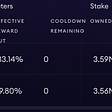# 56153 = 233 x 241

Government and military labs covet quantum computers because these devices can easily find the prime factors of large numbers. That’s hugely important for cracking the current generation of cryptographic codes.

The problem, however, is that building powerful quantum computers is hard. Consequently, the world record for quantum factorisation is just 143 (11 x 13), a feat performed by a group of Chinese physicists in 2012.

These guys did the job with a nuclear magnetic resonance quantum computer that used just four qubits to find the answer. But the solution they found is more remarkable than they originally supposed, say Nikesh Dattani at the University of Oxford in the UK and Nathaniel Bryans at the University of Calgary in Canada.

Today, these guys show mathematically that the same quantum computation that factors 143 also factors an entire class of much larger numbers, such as 3599 and 11663. Indeed, they go on to show that the Chinese group actually factored the number 56153 (233 x 241). That’s the largest quantum factorisation ever performed by some margin.

So how did the Chinese team miss this? The answer lies in the nature of the quantum algorithm they used to perform the factorisation. The most famous quantum factorisation algorithm was developed by mathematician Peter Shor back in 1994 and was first used in 2001 to find the factors of the number 15.

Shor’s algorithm has a weakness, however. It requires 8 qubits to perform this factorisation, which is more than physicists can generally handle in a quantum computer. So Instead they’ve performed the factorisation with fewer qubits by relying on prior knowledge of the answer. Even with this trick, the largest number factored using Shor’s algorithm is only 21.

There is another way of doing it, however. This works when the factors differ by two, such as 11 and 13 as factors for 143. The trick is to write out the numbers in binary form but replace two of the digits with variables, then multiply them together and set the answer to 143.

This generates a set of equations relating these variables. And the smallest solution to this set of equations reveals the factors.

The difficult part, however, is to find this minimal solution. But it turns out that a quantum computer can do this with only 4 qubits, which is exactly the calculation that the Chinese team performed with their quantum computer in 2012 giving the answer 11 x 13.

The work that Dattani and Bryans have done is to show that exactly the same calculation works for much bigger numbers as well. They repeat the same process of writing out the multiplication table for bigger numbers and show that the resulting set of equations are identical to the ones produced for 143. “Other numbers that we have discovered reduce to these same equations include 3599, 11663, and 56153,” they say.

In fact, they’ve discovered a general rule that applies to the product of any two numbers that differ by only two.

The significance of this work is that it shows that the Chinese team not only factored the number 143 but also some much larger numbers including 56153, which has the factors 233 and 241 (which differ by two bits in binary).

It’s easy to imagine that government and military labs will be rubbing their hands together in glee at the news that such large numbers can be factored with such simple quantum computers. This glee would be misplaced.

Dattani and Bryans’ trick only works with numbers that have factors that differ by two. So when it comes to code breaking, this trick is of little use unless the code breaker knows in advance that the factors differ in this way, something that is highly unlikely.

And in any case, because this trick works using only 4 qubits, it can easily be reproduced on any classical computer. So it’s not so useful after all.

Nevertheless, the work shows that a little mathematics can vastly increase utility of quantum computers. The real problem, of course, is to build ones that work with hundreds of qubits and not just a handful.

Ref: arxiv.org/abs/1411.6758 : Quantum Factorization Of 56153 With Only 4 Qubits

--

--

--

## More from The Physics arXiv Blog

An alternative view of the best new ideas in science. About: http://tinyurl.com/p6ypk56## The Physics arXiv Blog

An alternative view of the best new ideas in science. About: http://tinyurl.com/p6ypk56

## More from Medium